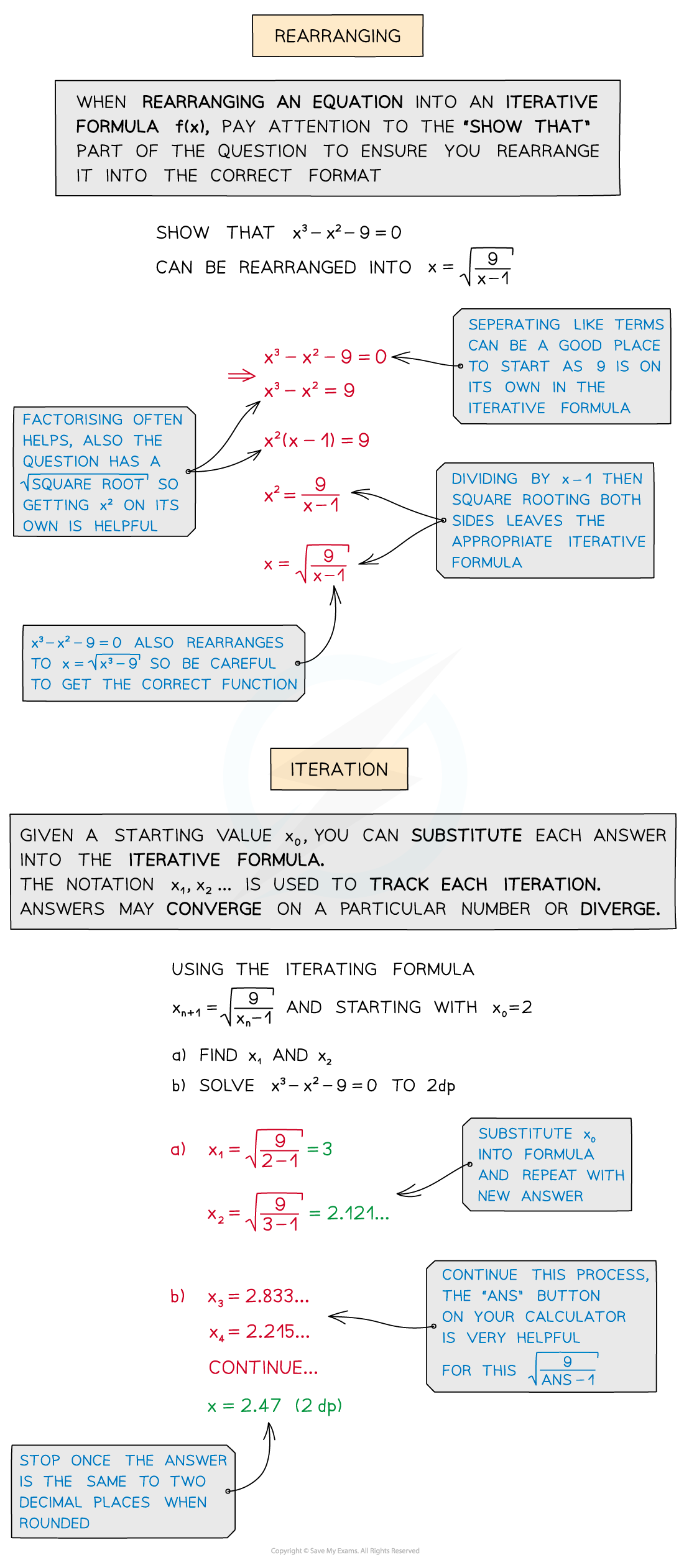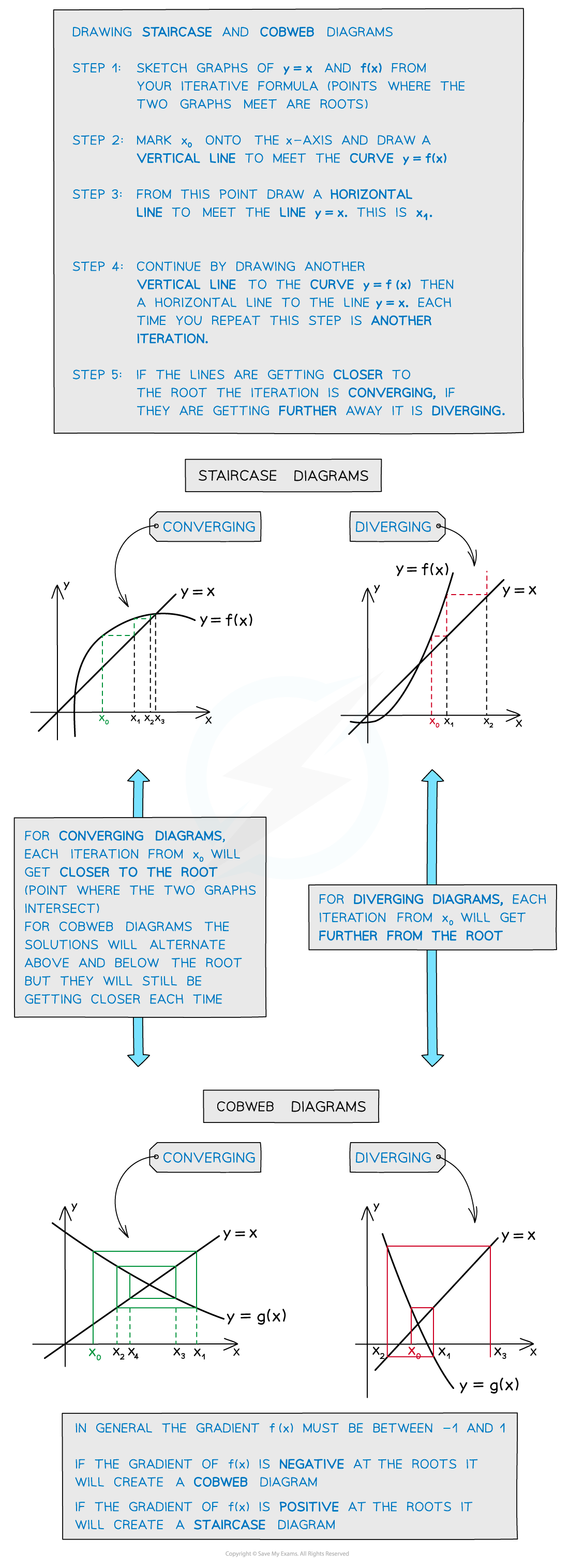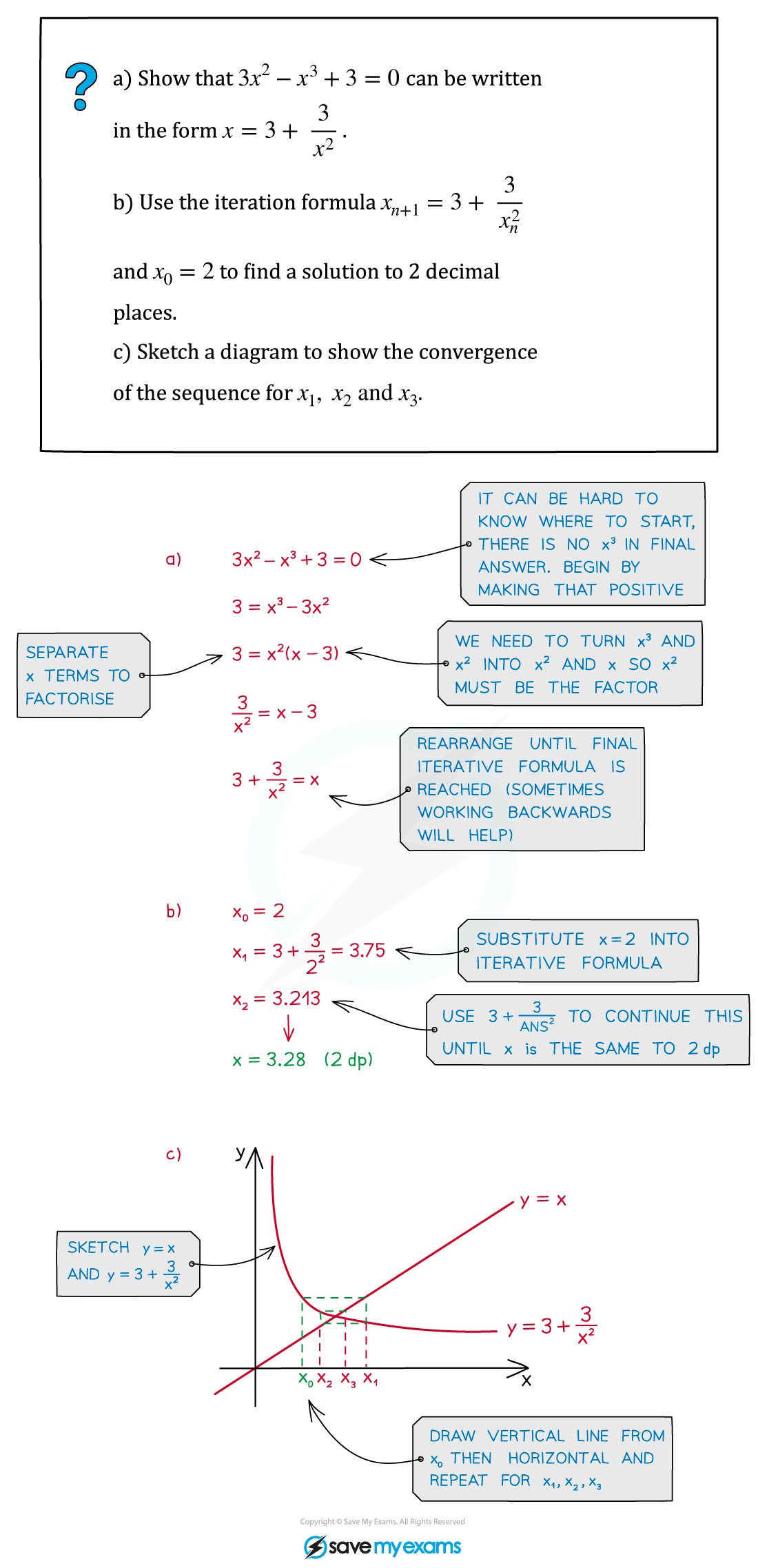# CIE A Level Maths: Pure 3复习笔记6.1.3 x = g(x) Iteration

### x = g(x) Iteration

#### What does xn + 1 = f(xn) mean?

• When an equation cannot be solved using the usual analytical methods, we can still find approximate solutions to a certain degree of accuracy
• Iteration is one way to do this, by repeatedly using each answer as the new starting value for a function, we can achieve an ever more accurate answer
• Iterations are shown using the notation xn + 1 = f(xn)
• This is a recurrence relation where, starting with a number (xn), we will get an answer xn + 1 which we can then reuse in the original function
• Equations need to be rearranged into an iterative formula – ie. the form x = f(x)#### Iteration diagrams

• Iterations can be shown on diagrams called staircase or cobweb diagrams
• These can be drawn by plotting the graphs of y = x against y = f(x) from your iterative formula#### Exam Tip

• You must show all your steps when rearranging an equation into an iterative formula
• Working backwards can often be helpful to figure out how an equation has been rearranged but you must write your answer as if you worked forwards
• Use ANS button on your calculator to calculate repeated iterations
• Keep track of your iterations using x2, x3… notation
• Iteration may be part of bigger numerical methods questions

#### Worked Example